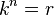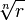# Nth root facts for kids

Kids Encyclopedia Facts

Nth root of a number r is a number which, if multiplied by itself n times, makes r. You could also say that it is a number k for which the following equation is true:$k^n=r$

(for meaning of$k^n$, read exponentiation.)

We write it like this:$\sqrt[n]{r}$.Nth root Facts for Kids. Kiddle Encyclopedia.